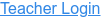PhET Interactive Simulations

### Topics

• Gravitational Force
• Newton's Third Law
• Force Pairs
• Force
• Gravity

### Description

Visualize the gravitational force that two objects exert on each other. Adjust properties of the objects to see how changing the properties affects the gravitational attraction.

### Sample Learning Goals

• Relate gravitational force to masses of objects and distance between objects.
• Explain Newton's third law for gravitational forces.
• Design experiments that allow you to derive an equation that relates mass, distance, and gravitational force.
• Use measurements to determine the universal gravitational constant.
Version 2.1.3